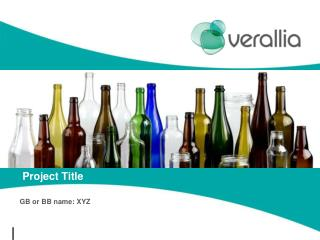DownloadDownload PresentationProject Title

# Project Title

Download Presentation## Project Title

- - - - - - - - - - - - - - - - - - - - - - - - - - - E N D - - - - - - - - - - - - - - - - - - - - - - - - - - -
##### Presentation Transcript

1. Project Title GB or BB name: XYZ

2. Define Phase C I A M D

3. D M A I C Project Charter

4. D M A I C Framing Worksheet

5. D M A I C CustomerRequirements CTQ’s

6. D M A I C SIPOC For example

7. D M A I C Stakeholder Analysis Who are they? Where are they? Where do we need them to be? How do we get them there? Who could help?

8. D M A I C ROSS Business case

9. D M A I C Measure phase C D M I A

10. D M A I C Current process map Include some initial map of your process

11. D M A I C Ys behavior

12. D M A I C Data collection Pareto Check sheet

13. D M A I C Measurement System Analysis Is the Measurement System adequate ______ Yes/No Potential source(s) of measurement variation checklist Yes/No Evidence Is my measurement system repeatable? _______ Will I get the same results if I make the measurement more than once? Is my measurement system reproducible? _______ Will someone else be able to complete the same measurement & get the same results? Is my measurement system accurate? _______ Will the results from the study match the actual value or expert data? The appropriate analysis tool to quantify the amount of variation due to the measurement system is _______ Very simple assessment _______ Discrete data analysis tool (for repeatability, reproducibility & accuracy) _______ Gage R&R - ANOVA method _______ Gage R&R - Short method Only if issues on Measuring system

14. D M A I C Gage R&R • Set up your table for measure like this: • Go to Stat/quality tool/Gage study/gage R&R study (Crossed) • ANOVA or Xbar and R and run • You will get something like this

15. D M A I C D N x O Baseline process sigma (method 1) • Number of units processed N = __________ • Total number of defects made (include defects made and later fixed) D = __________ • Number of defect opportunities per unit (equate to CTQs) O = __________ • Calculate # defects per million opportunities DPMO = 1,000,000 x ( ) = 1,000,000 x = __________ • Look up process sigma in Sigma = __________ sigma conversion table ( ) ( ) ( )

16. D M A I C Baseline process sigma (method 2) • short term sigma = • long term sigma value = • Stable or unstable ?

17. D M A I C Analyse phase C D M I A

18. D M A I C Cause & effect diagram

19. D M A I C 5 Whys Analysis  Catalyst Consulting Ltd. 2006

20. D M A I C Minitab Analysis  Catalyst Consulting Ltd. 2006

21. D M A I C Y=f(Xi)

22. D M A I C Vital few Causes

23. D M A I C Improve phase I C A D M

24. D M A I C Proposed solution

25. ACTION PLAN Saint-Gobain Containers

26. D M A I C Stakeholder Analysis - improve Who are they? Where are they? Where do we need them to be? How do we get them there? Who could help?

27. D M A I C Control phase C I A D M

28. D M A I C Control Plan

29. D M A I C Final process sigma (method 1) • Number of units processed N = __________ • Total number of defects made (include defects made and later fixed) D = __________ • Number of defect opportunities per unit (equate to CTQs) O = __________ • Calculate # defects per million opportunities DPMO = 1,000,000 x ( ) = 1,000,000 x = __________ • Look up process sigma in sigma conversion table Sigma = __________ D N x O ( ) ( ) ( )

30. D M A I C Final process sigma (method 2) • Stable or unstable ? • short term sigma = • long term sigma value =

31. KPI SPC Before After Saint-Gobain Containers

32. D M A I C ROSS Control – the final outcome